# Student Projects

cancel
Showing results for
Did you mean:

# Contact Information

University: Harbin Institute of Technology

Team Members (with year of graduation): Pingfan Song, Jianing Zhang, Aiqiang Lei,Congru Yin, Tingting Yao

Submission Language: English

# Project Information

Description:

ADC's high sampling rate brings us too much redundant data. For example, our camera generally preserves 10% total data and discards the remaining 90% after compression. AIC is a new technique that different with ADC. AIC converts analog signal to valuable digital information that we just need. By this way, we can break though the limit of Nyquist sampling theorem and significantly reduce the sampling rate and data volume. Our design is a showcase of AIC's wonderful effect.

Products

PXIe1082, PXIe-PCIe8375, LabVIEW 2011 and some devices driver (NIDAQ...)

The Challenge

How to reduce the sampling rate of multi-tone analog signal and data volume? How to synchroize multiple tasks effectively?

The Solution

As shown in Figure 1, x(t) is an input analog signal which is sparse in frequency domain. We utilize the ao function of PXIe-6368 to output the test signal. pc(t) is a pseudo-random sequence with values of {±1} and it alternates between values at or faster than the Nyquist frequency of the input signal x(t). We utilize the ao function of PXIe-5442 to output the sequence. x(t) is multiplied by pc(t) in a mixer or multiplier. Multiplying a signal with another in time domain means convoluting their spectrums in frequency domain. In this way, input signal’s spectrum is spread and every point in frequency domain contains all the information of the input signal. An analog low-pass filter follows the mixer and then a traditional ADC samples the output signal at low sampling rate. Finally, the input signal is reconstructed in computer from the sampled data using some optimization algorithms.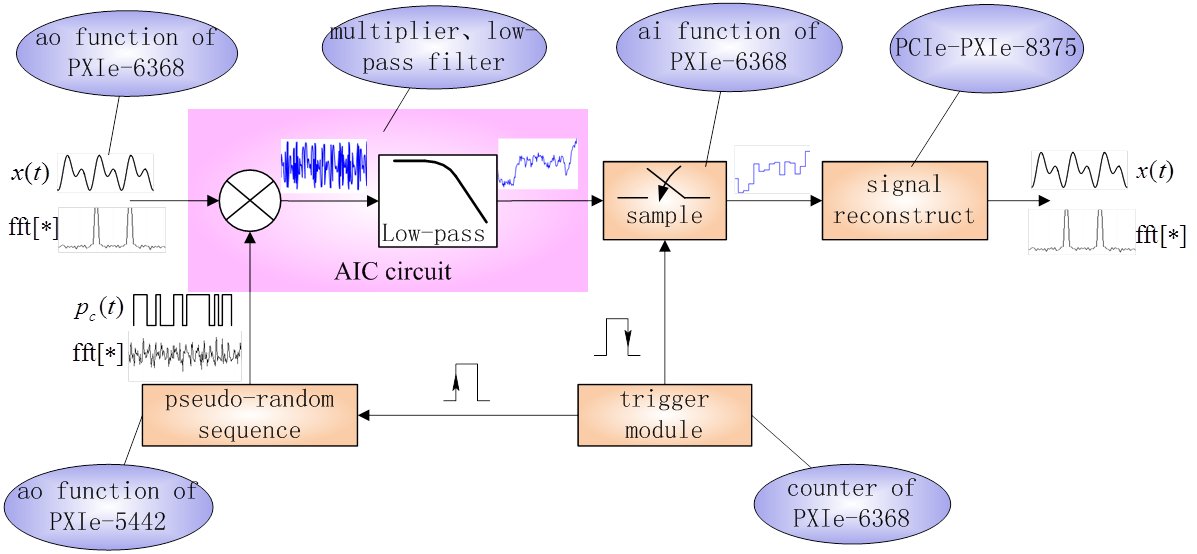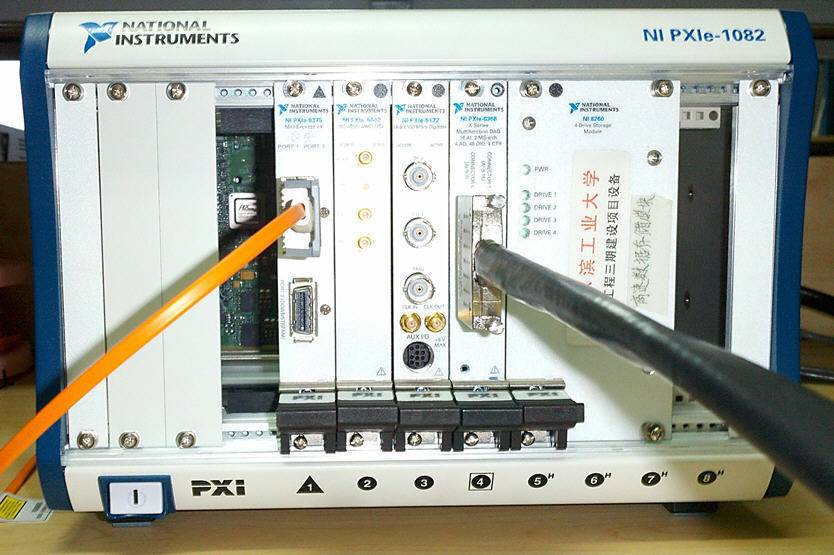figure 1 AIC frame and hardware system

<Explain the benefits using LabVIEW and NI tools.>

LabVIEW and NI tools help us accomplish our project more quickly and more effectively. And the experiment results are more credible.
<Insert image(s) of project with captions.>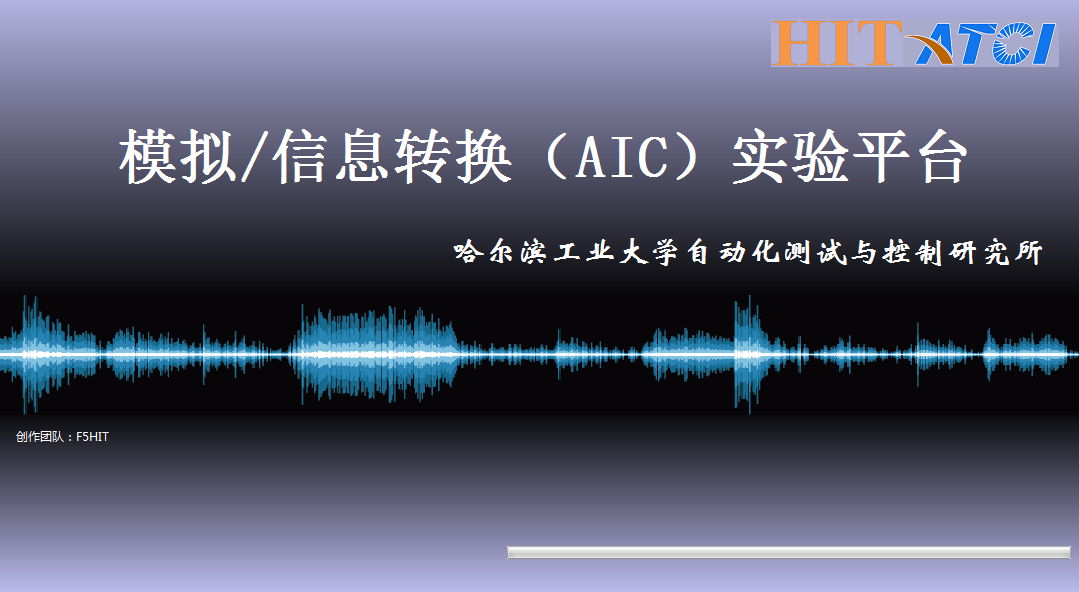figure 2 startup screen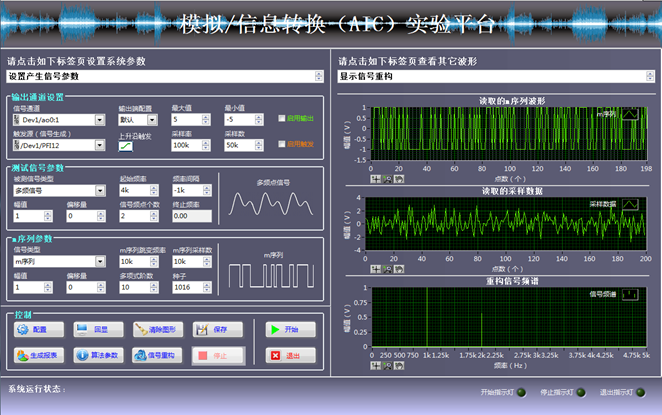figure 3 main screen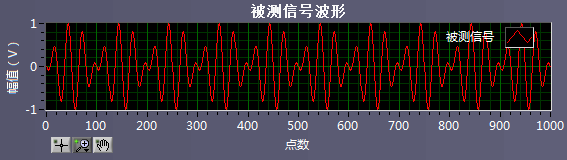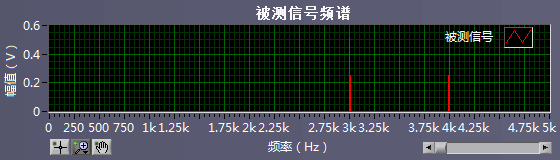figure 4 the wave and spectrum of an original signal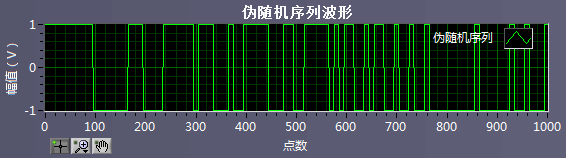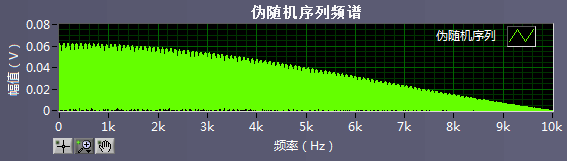figure 5 the wave and spectrum of a pseudo-random sequence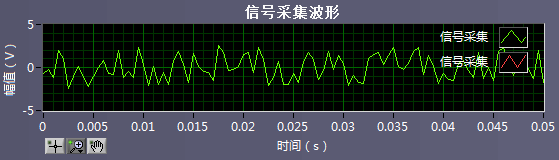figure 6 sampled data with 2kS/s sampling rate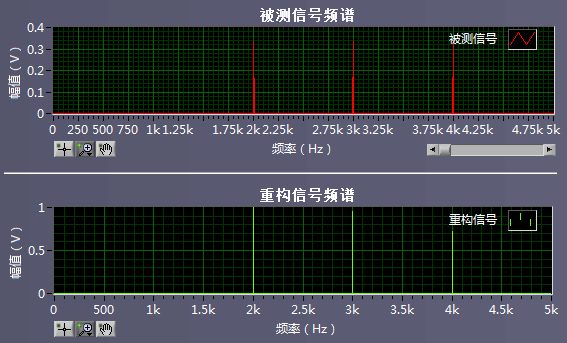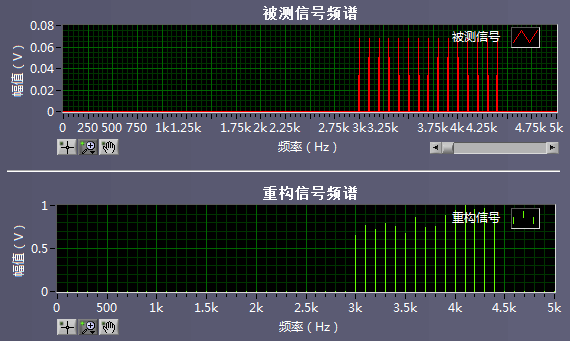figure 7 the spectrum of original signals and reconstructed signals

<Insert video here.>

<Attach VI code (optional).>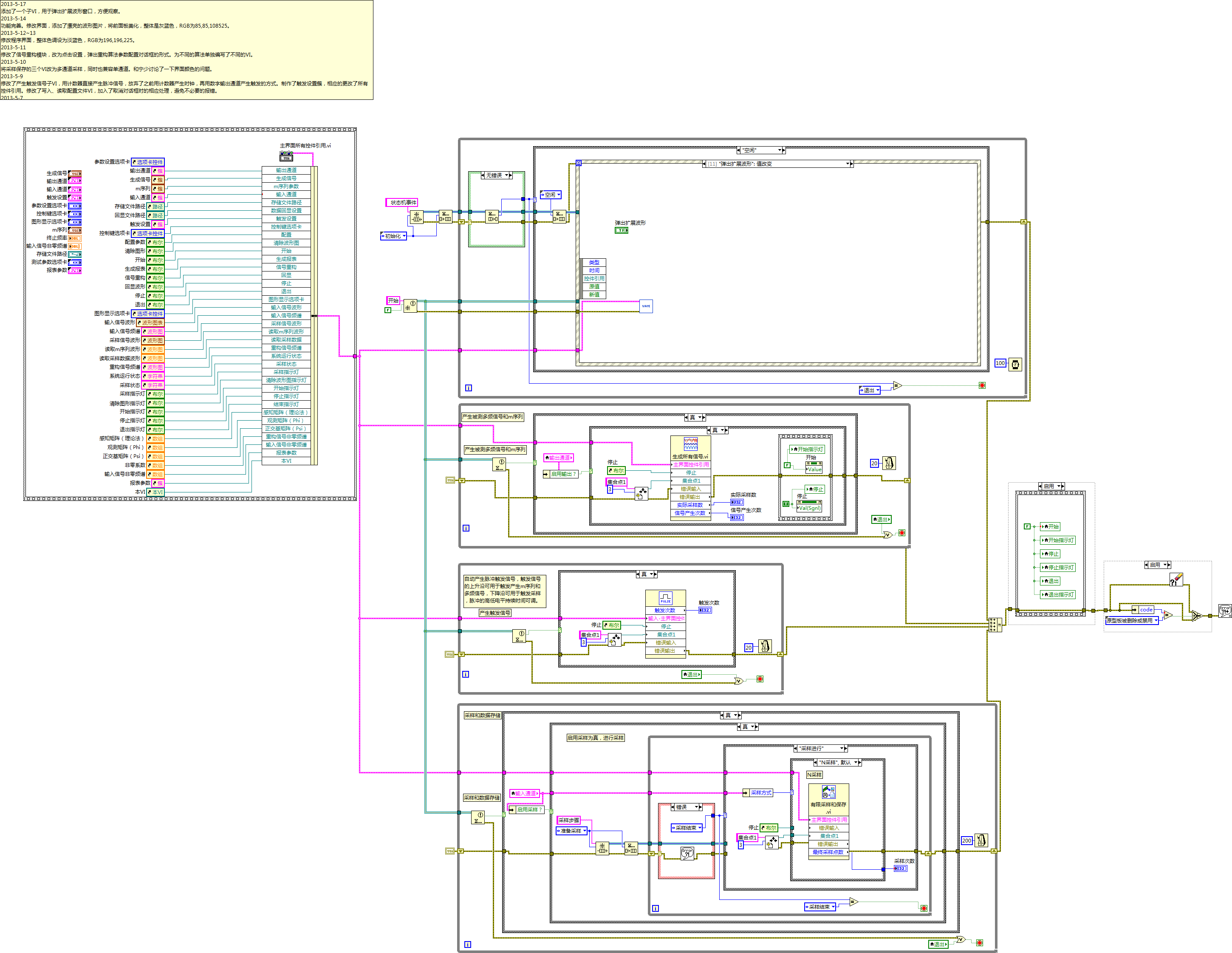figure 8 the main code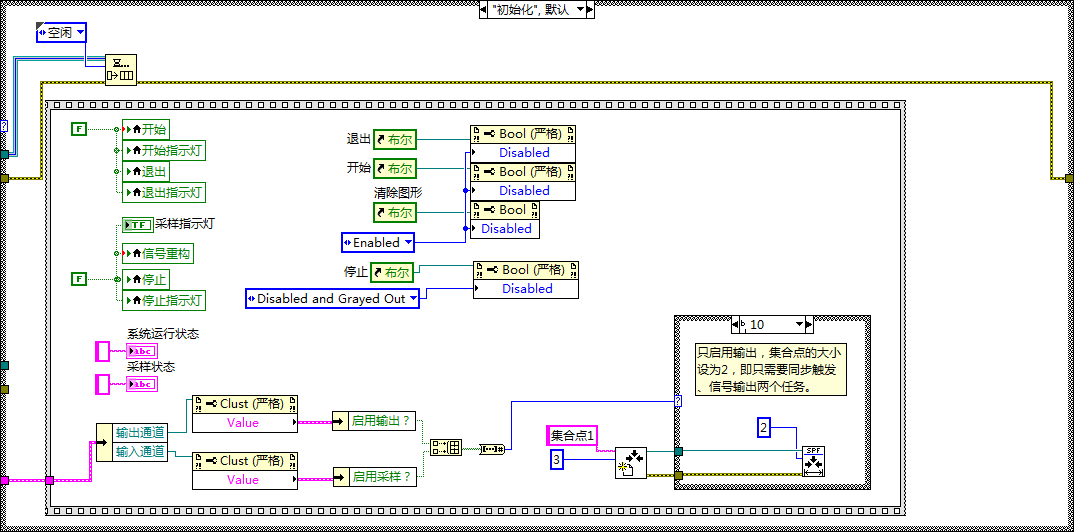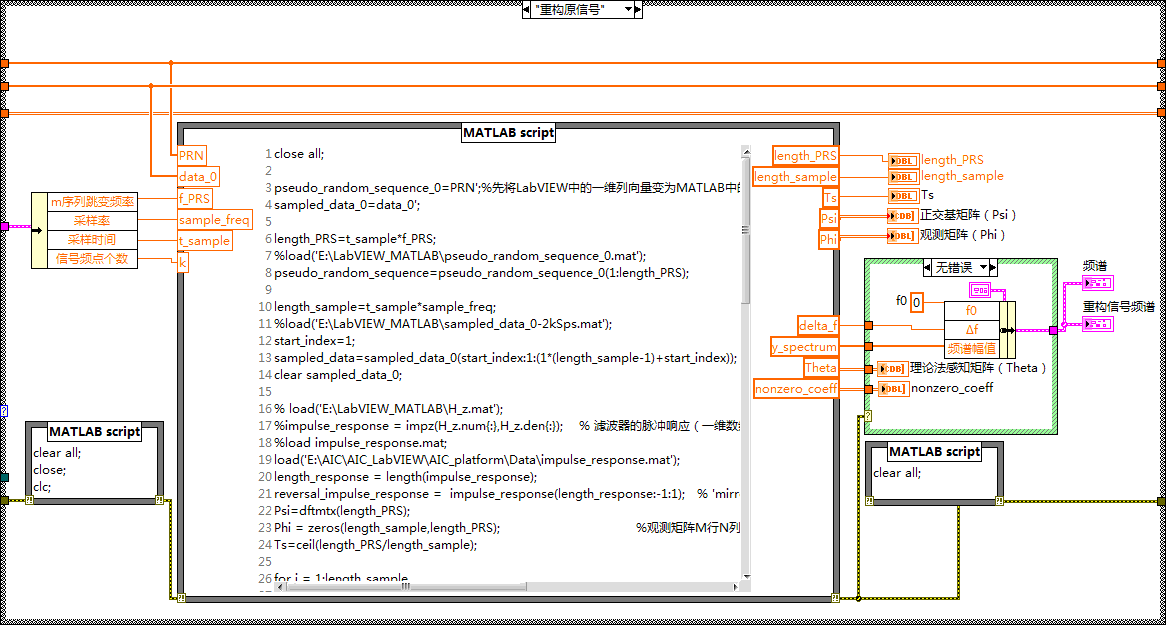figure 9 the codes of a part of VIs

<Level of completion (beta, alpha, or fully functional)>

<Time to build>